Next: SLA_TPV2C - Plate centre from and x,y,z
Up: SUBPROGRAM SPECIFICATIONS
Previous: SLA_TP2V - Tangent Plane to Direction Cosines

## SLA_TPS2C - Plate centre from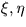and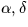ACTION:
From the tangent plane coordinates of a star of known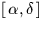,determine theof the tangent point (single precision)

CALL:
CALL sla_TPS2C (XI, ETA, RA, DEC, RAZ1, DECZ1, RAZ2, DECZ2, N)

GIVEN:

 R XI,ETA tangent plane rectangular coordinates (radians) RA,DEC R spherical coordinates (radians)

RETURNED:

 R RAZ1,DECZ1 spherical coordinates of tangent point, solution 1 RAZ2,DECZ2 R spherical coordinates of tangent point, solution 2 N I number of solutions: 0 = no solutions returned (note 2) 1 = only the first solution is useful (note 3) 2 = there are two useful solutions (note 3)

NOTES:
1.
The RAZ1 and RAZ2 values returned are in the range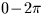.
2.
Cases where there is no solution can only arise near the poles. For example, it is clearly impossible for a star at the pole itself to have a non-zero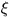value, and hence it is meaningless to ask where the tangent point would have to be to bring about this combination ofand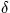.
3.
Also near the poles, cases can arise where there are two useful solutions. The argument N indicates whether the second of the two solutions returned is useful. N=1 indicates only one useful solution, the usual case; under these circumstances, the second solution corresponds to the over-the-pole'' case, and this is reflected in the values of RAZ2 and DECZ2 which are returned.
4.
The DECZ1 and DECZ2 values returned are in the range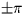, but in the ordinary, non-pole-crossing, case, the range is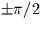.
5.
RA, DEC, RAZ1, DECZ1, RAZ2, DECZ2 are all in radians.
6.
The projection is called the gnomonic projection; the Cartesian coordinates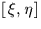are called standard coordinates. The latter are in units of the distance from the tangent plane to the projection point, i.e. radians near the origin.
7.
When working in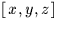rather than spherical coordinates, the equivalent Cartesian routine sla_TPV2C is available.Next: SLA_TPV2C - Plate centre from and x,y,z
Up: SUBPROGRAM SPECIFICATIONS
Previous: SLA_TP2V - Tangent Plane to Direction Cosines

SLALIB --- Positional Astronomy Library